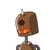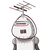# A bag contain 3white and 2red balls. one ball is drawn in random. what is the probability that the ball drawn is red​

A bag contain 3white and 2red balls. one ball is drawn in random. what is the probability that the ball drawn is red​

### 2 thoughts on “<br />A bag contain 3white and 2red balls. one ball is drawn in random. what is the probability that the ball drawn is red​”

1.3 white balls and 2 red ball it means that total balls are 5

and red balls are 2

p(e)=no.of trials in which the event occured/total outcome

## =2/5

2.